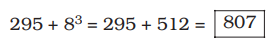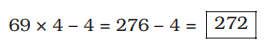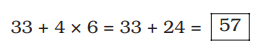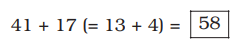Q:

She broke down in the middle of her speech.

a

could not proceed

b

fell down

c

became angry

d

cried

Explanation :

Phrase Break down means : to lose control of your feelings and start crying.

### Related Questions

1.

7 15 79 295 ? 1807

a

815

b

807

c

823

d

829

e

795View Answer ||Discuss
Explanation :
The pattern is : 7 + 23 = 7 + 8 = 15 $15+4^{3}=15+64=79$ $79+6^{3}=79+216=295$ /$807+10^{3}=807+1000=1807$

2.

14 13 24 69 ? 1355

a

272

b

284

c

276

d

264

e

268View Answer ||Discuss
Explanation :
The pattern is : 14 × 1 – 1 = 14 – 1 = 13 13 × 2 – 1 = 26 – 2 = 24 24 × 3 – 3 = 72 – 3 = 69272 × 5 – 5 = 1360 – 5 = 1355

3.

7 7 10 18 33 ?

a

65

b

57

c

69

d

55

e

67View Answer ||Discuss
Explanation :
The pattern is : 7 + 0 × 2 = 7 + 0 = 7 7 + 1 × 3 = 7 + 3 = 10 10 + 2 × 4 = 10 + 8 = 18 18 + 3 × 5 = 18 + 15 = 334.

3 10 18 28 41 ?

a

54

b

58

c

56

d

62

e

60View Answer ||Discuss
Explanation :
The pattern is : 3 + 7 = 10 10 + 8 (= 7 + 1) = 18 18 + 10 (= 8 + 2) = 28 28 + 13 (= 10 + 3) = 415.

Kajal spends 55% of her monthly income on grocery, clothes and education in the ratio of 4 : 2 : 5 respectively. If the amount spent on clothes is 5,540, what is Kajal’s monthly income ?

a

55,650

b

55,400

c

54,500

d

55,450

e

None of theseView Answer ||Discuss
$x imes frac{55}{100} imes frac{2}{(4+2+5)}=5540$
$Rightarrow x imes frac{55}{100} imes frac{2}{11}=5540$
$Rightarrow x=frac{5540 imes 100 imes 11}{55 imes 2}$
$=$ Rs. 55400